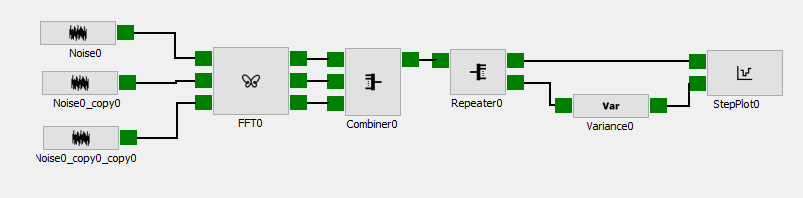Version: current

# Variance

## Description#

The statistics block `variance` calculates the variance of a dataset on either axis 0 or 1.

#### Input#

The input is `MxN`.

#### Output#

The output is a variance of the data on either axis 0 or 1. if the variance is calculated across axis 0 the output is `1xL`, or if the variance is calculated across axis 1 the output is `Kx1`.

## Attributes#

AttributeValue
NameVariance
Axis0
Channels1

#### Name#

Name specifies the name of the block.

#### Axis#

Axis specifies the axis of the dataset where the variance should be calculated.

#### Channels#

Channels specifies the number of input(s)/output(s) the block will have.

## Examples#

This example 3 pink noise signal with different amplitudes, and the variance the three noise signals at each frequency point.